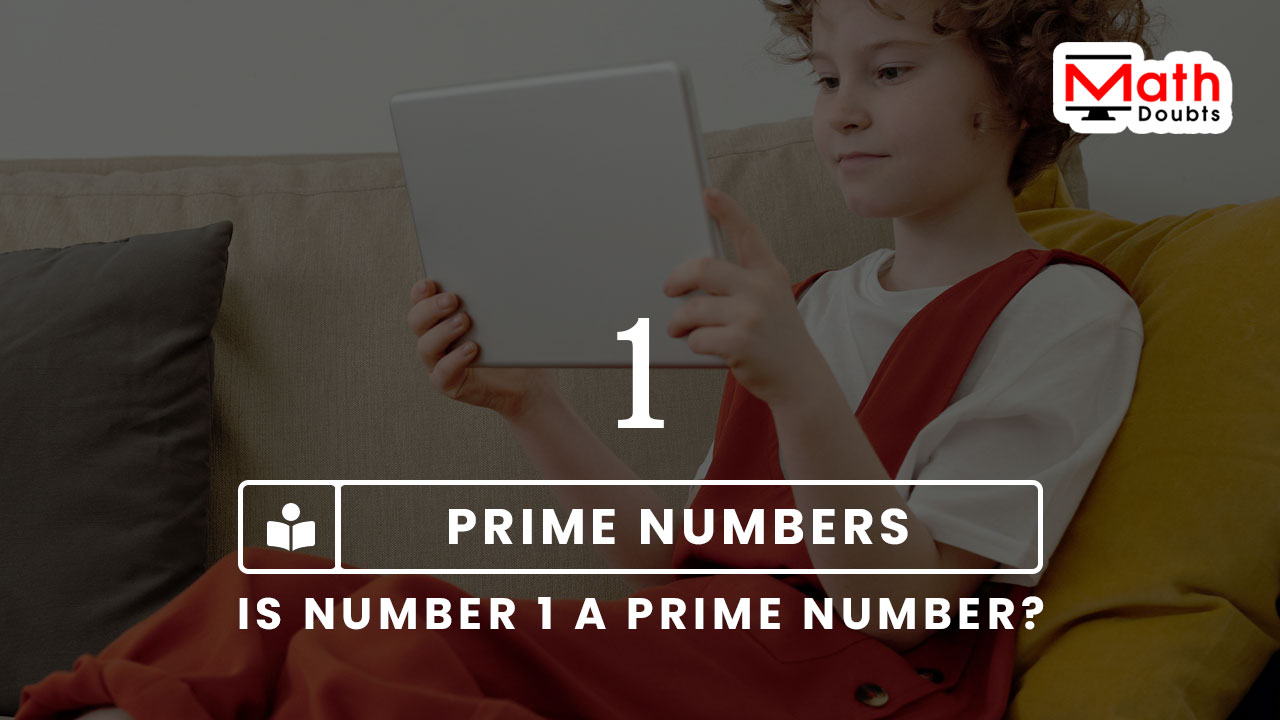# Is 1 a Prime number?

One is a first natural number and let’s determine whether the natural number $1$ is a prime number or not by the fundamental definition of a prime number.According to the definition of a prime number, firstly divide the natural number $1$ by the one.

$1 \div 1$

$\implies$ $\dfrac{1}{1} \,=\, 1$

The natural number $1$ is completely divisible by the $1$. So, the quotient of $1$ divided by $1$ is $1$. It clears that there is a possibility for the natural number $1$ to become a prime number.

However, the natural number $1$ is not a prime number because the natural number $1$ should be divided by one and itself but they both are same in this case. It can be observed by writing the natural number $1$ in factor form.

$\,\,\,\therefore\,\,\,\,\,\,$ $1 \,=\, 1 \times 1$

The natural number $1$ is not following the definition of a prime number strictly. For that reason, the natural number $1$ is not considered as a prime number.

###### Recommended
Latest Math Topics
Jun 26, 2023
Jun 23, 2023

Latest Math Problems
Jul 01, 2023
Jun 25, 2023
###### Math Questions

The math problems with solutions to learn how to solve a problem.

Learn solutions

Practice now

###### Math Videos

The math videos tutorials with visual graphics to learn every concept.

Watch now

###### Subscribe us

Get the latest math updates from the Math Doubts by subscribing us.